# Kullback-Leibler information

Kullback–Leibler quantity of information, Kullback–Leibler information quantity, directed divergence

For discrete distributions (cf. Discrete distribution) given by probability vectors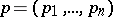,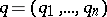, the Kullback–Leibler (quantity of) information ofwith respect tois:whereis the natural logarithm (cf. also Logarithm of a number).

More generally, one has: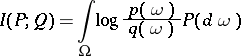for probability distributionsandwith densities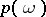and(cf. Density of a probability distribution).

The negative of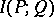is the conditional entropy (or relative entropy) ofwith respect to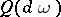; see Entropy.

Various notions of (asymmetric and symmetric) information distances are based on the Kullback–Leibler information.

The quantity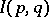is also called the informational divergence (see Huffman code).